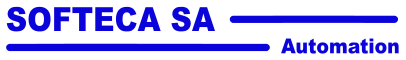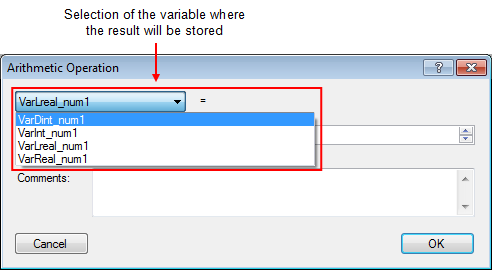# Presse universelle MecaMotion parNavigation: Part program instructions Arithmetic operations

Arithmetic operations

In this section, you will find the different arithmetic operations that you can perform in the part-program.

Below is a list of the instruction's input and output parameters:

Parameter name

Declaration

Type of data

Default value

Description

Result

Output

REAL, LREAL, INT ou DINT

--

Variable where the result of the operation is stored

Operand 1

variable or constant

Input

REAL, LREAL, INT ou DINT

--

First operand (variable or constant)

Operand 2

variable or constant

Input

REAL, LREAL, INT ou DINT

--

Second operand (variable or constant)

Type of operation

Input

"+", "-", "/", "x"

"+"

Type of operation, addition, subtraction, division, multiplication

Table 1: List of instruction parameters

The result of an arithmetic operation will always be stored in a user variable. This variable can be of any format (see figure 1).Figure 1: User variable selection containing operation result

In figure 1, the variable containing the result can be declared in the formats below:

INT (16 bits)

DINT (32 bits)

REAL (32 bits floating)

LREAL (64 bits floating)

These different operations can be done between different variable formats, for example the addition of a INT and a REAL and the result is returned in LREAL format.

Internally, each operand, whatever its format, is automatically transformed into LREAL (64 floating bits), all calculations are performed in this format. When the calculation is performed, the result is converted to the format of the result variable.

If the result is to be stored in a user variable of type INT, and the result is greater than 32760 or smaller than 32760, then error n°16 ("arithmetic overflow on integer") occurs. The execution of the program is then stopped.

When the result is to be transferred to a user variable of type DINT, the same error occurs if the result exceeds 2000000000 or -2000000000.

Below is a list of the arithmetic operations that can be performed in the part-program: